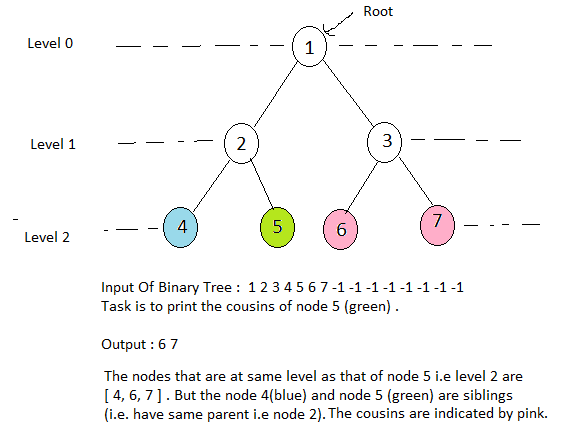# Cousins of Given Node in Binary Tree

Posted: 12 Nov, 2020
Difficulty: Moderate

## PROBLEM STATEMENT

#### Given a binary tree of N nodes and a node of this tree, you need to return a list containing the values of the cousins of the given node in the given binary tree sorted by non-decreasing order of their values.

##### Note:
``````Two nodes of a binary tree are cousins if they have the same depth or level, but have different parents.

No two nodes in the given binary tree will have the same data values.
``````
##### Example :##### Input Format:
``````The first line contains a single integer T representing the number of test cases.

The first line of each test case will contain the values of the nodes of the tree in the level order form ( -1 for NULL node). Refer to the below example for further clarification.

The second and the last line of each test case will contain the value of the node for which the cousins are to be found.

Example:
Consider the binary tree:

The input of the tree depicted in the image above will be like:

1
2 3
4 -1 5 6
-1 7 -1 -1 -1 -1
-1 -1

Explanation :
Level 1 :
The root node of the tree is 1

Level 2 :
Left child of 1 = 2
Right child of 1 = 3

Level 3 :
Left child of 2 = 4
Right child of 2 = null (-1)
Left child of 3 = 5
Right child of 3 = 6

Level 4 :
Left child of 4 = null (-1)
Right child of 4 = 7
Left child of 5 = null (-1)
Right child of 5 = null (-1)
Left child of 6 = null (-1)
Right child of 6 = null (-1)

Level 5 :
Left child of 7 = null (-1)
Right child of 7 = null (-1)

The first not-null node (of the previous level) is treated as the parent of the first two nodes of the current level. The second not-null node (of the previous level) is treated as the parent node for the next two nodes of the current level and so on.

The input ends when all nodes at the last level are null (-1).
``````
##### Note :
``````The above format was just to provide clarity on how the input is formed for a given tree.

The sequence will be put together in a single line separated by a single space. Hence, for the above-depicted tree, the input will be given as:

1 2 3 4 -1 5 6 -1 7 -1 -1 -1 -1 -1 -1
``````
##### Output format :
``````For each test case, print the value of the cousins of the given node in the binary tree, or -1 if the given node has no cousins in the binary tree.
``````
##### Note:
``````You do not need to print anything, it has already been taken care of. Just implement the given function.
``````
##### Constraints:
``````1 <= T <= 100
1 <= N <= 3000
1 <= nodeVal <= 10^9

Time Limit: 1 sec
``````Approach 1
• Find the level of the given node of the binary tree. For this we will use a recursive approach, i.e. DFS. Keep in mind that the level of a parent is 1 less than its children.
• Store all the nodes at the same level of the given node i.e. we found in the previous step in array.
• Traverse array and remove those nodes which are siblings to the given node i.e. (parent of given node and node in array is the same.) .
• Return the list.